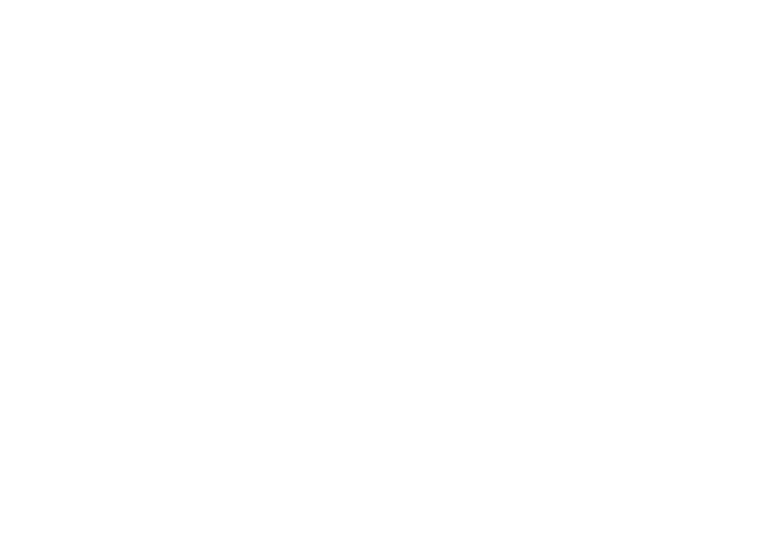# There are only 10 types of people in the world…##### A Quick Introduction to Basal Numbers

Our number system has evolved a lot over the years, but for a while now, we have established the base 10 number system (the decimal system) as being the norm. It’s great because calculation becomes very easy due to it being something our brains can deal with quite efficiently.

Base 10 means that each position in a written number represents a quantity of a specific power of 10. We start from the right hand side of the number. The final digit represents the amount of 100, the next one to the left represents the amount of 101, the next 102 and so on. This is also why we have 10 different digits – we don’t need a symbol for ten after nine because you just move to the next column.

For example, 2431 is 1×100; + 3×101 + 4×102 + 2×103, which is 1 + 30 + 400 + 2000. This is simple – you would have learnt it when you first learnt how to count.

However, numbers can be written to any base. Usually, it’s pretty pointless to do so because they become very difficult for us to work with – and for bases greater than 10, we have to create more symbols to represent the additional numbers (base 11 would need an extra symbol for 10 etc.)

But systems other than the decimal system are still used. By far the most common is base 2 – or binary. Binary numbers only need 2 symbols to represent numbers – 0 and 1. This is extremely useful in the world of electronics. Because electricity (and hence anything digital) can only be on or off, there are only 2 different signals available which we must use to represent a vast amount of different information. This is where binary comes into immense usefulness.

##### Binary to Decimal

The concept is still the same as the decimal system, but now we are working with powers of 2 rather than 10.

Say we see the binary number 100110. What is it in decimal format?

Following the same logic as before and working from right to left, we have 0x20 + 1×21 + 1×22 + 0x23 + 0x24 + 1×25 = 0 + 2 + 4 + 0 + 0 + 32 = 38.

So 100110 in binary is equal to 38 in decimal. Easy-peasy.

##### Quotients and Remainders

Before I convert a decimal number to a binary number, I need to show you the concept of a quotient and remainder (if you don’t know this already). When we want to divide a number by another, sometimes we get a whole answer. This is the quotient. But most of the time, we won’t be able to divide exactly. In this case, we find the highest amount of times that the number goes into the other (this is still the quotient) and add on whatever is left over. The left-over amount is called the remainder. We are not using fractions or decimals at all – so pretend they don’t exist.

To simplify this, suppose we have a number “x”. Then regardless of what x is, we can write it in the following form:

x = qd + r

Here, q is called the quotient, r is called the remainder, and d is the number that you want to divide x by. For example, take x=34 and d=6. Then x = 5*6 + 4 (the asterisk represents multiplication to prevent confusion with the value x).

##### Decimal to Binary

Now, suppose we want to convert 85 into binary. Using the quotient and remainder method, we set the divisor to be 2 (because we are in base 2). This always stays the same.

If we divide 85 by 2, we get 42 and 1 left over. You can easily do this in your head if you wish, but I will write it out:

85 = 42*2 + 1

Take note of the remainder. Now we take our quotient as the new “x” and repeat the process:

42 = 21*2 + 0
21 = 10*2 + 1
10 = 5*2 + 0
5 = 2*2 + 1
2 = 1*2 + 0
1 = 0*2 + 1

Here we stop, because the quotient has become 0. If we carry on, we get an endless number of lines that look like: 0 = 0*2 + 0 which is not that useful to us.

We’re done! All that needs to be done is to write the remainders in order – but make sure the first remainder goes on the right, the next goes to its left and so on.

This gives us 1010101 (that’s quite pretty – I didn’t actually plan that!) as being the binary form for 85. We can check this: 1 + 0 + 4 + 0 + 16 + 0 + 64 = 85.

———————————————

The beauty of these methods is that they can just as easily be used to convert a decimal number to and from any other basal form – just replace where I’ve used 2 with whichever base you like. The reason that I wrote the quotient and remainder part in a very specific way is to make it easier if you use a trickier base to work with – where doing it in your head isn’t quite as easy.

Have fun with it!